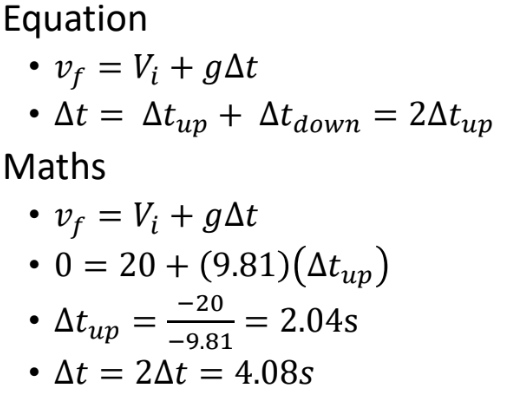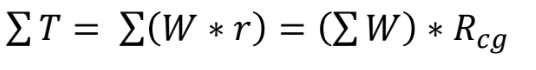# Biomenchanics exam #1 (Terms+others) Flashcards

Set Details Share
created 13 days ago by aireen_c
7 views
updated 11 days ago by aireen_c
Page to share:
Embed this setcancel
COPY
code changes based on your size selection
Size:
X

1

Force

Push or pull on an object

2

Internal forces

• Act within an object or system whose motion is under investigation
• Forces come in pairs (action and reaction)

3

Internal forces: tensile force

When pulling forced act on the ends of an internal structure

4

Internal force: compressive forces

When pushing forces act oj the ends of an internal structure

5

External forces

Act on an object as a result of its interaction with the environment surrounding it (when two objects touch)

6

Weight

W = m*g

w (newtons)= m(in kilograms)*g(9.81m/s^2)

7

Translation:

linear motions in which all parts of a rigid body move parallel to & in the same direction as every other part of the body

8

Rotation:

• motion in which a (assumed) rigid body moves in a circular path around some pivot point,
• thus the entire body moves in the same angular direction & across the same number of degrees

9

Kinematics can be_____

active or passive

10

Degrees of freedom:

the number of independent directions of movements allowed at a joint

11

max degrees of angular freedom

3 degrees for 2 cardinal planes

12

Arthrokinematics: the trio

1. roll
2. slide
3. spin

13

Roll-slide

• As primary movement of bone is to rotate across another bone’s surface
• concurrent but equal + opposite side

14

spin

• Spinning of one articular surface on another
• NO SLIDE

15

THE RULE: Convex-on-concave =

opposite

16

THE RULE: Concave-on-convex

same

17

Joint articulation fits “the best” where?

• at end range = close packed
• provide more stability

18

Kinetics

The study of mechanics that describes the effect of forces on the
body

19

Rectilinear:

• all points on a body/object move in a straight line.
• Direction of motions& orientation of object do not change.
• All points on object move the
same distance.

20

Curvilinear:

• all points on a body or object move
• orientation does not
change & all points move the same distance
• path the object follows is
curved.

21

Angular motion

• rotation/rotary motion
• More numerous than linear motion (human movement)

22

General motion

• Combination of angular motions producing linear motions of multiple body parts

23

Displacement

• vector quantity
• has direction & magnitude

24

Speed

• rate of motion
• AVG= d/change in time

25

Velocity

• rate of motion in a specific direction
• AVG= velocity(or d)/change of time

26

Baseball fastpitch example

- baseball released @16.8m

- velocity @47.162m/s

• v= d/change of time
• change of t= d/velocity
• 16.8m/47.163(m/s)= 0.356 seconds

27

hang time when initial vertical velocity (20 m/s) and initial horizontal velocity (15 m/s) is known28

L = mv

• L = linear momentum
• m = mass
• v = instantaneous velocity
• L constant => sum of forces = 0

29

elastic collisions

momentum is conserved (transferred) and no energy is lost

30

inelastic collisions

momentum is conserved but energy is lost

31

Impulse

the product of force and the time the force acts

32

Torque equations

• T= F*r
• F = force
• r = moment arm (perpendicular distance from axis of rotation)

33

Stability

• dependent upon height of center of gravity, size of base of support, and the weight of an object

34

Locating Center of gravitysum of force

35

centric forces

act through the center of gravity of an object and cause linear translation

36

Eccentric forces

• (not muscle contractions) do not act through the center of gravity
• they cause both linear and angular motion

37

Force couples

• create angular motion
• couples are forces that
are equal in size, non-colinear, and act in opposite directions

38

1st class levers

• HUGE forces
• distance is really small.

39

2nd class levels

• middle
• output forces are modest, input distance is modest

40

3rd class:

• WEAK output forces
• HUGE distances

41

Potential Energy (2 types)

• Gravitational
• Strain (or spring) energy

42

Power

• strongest contraction force is produced at the slowest speed
• max POWER occurs at 1/3 -1/2 of a muscles max contraction velocity

43

Energy

the capacity to do work

44

Tension

the stress that acts perpendicular (or normal) to the analysis plane

45

Shear

A transverse stress that acts parallel to the plane of analysis

46

Bending

• More complicated
• creates different stresses at the analysis
plane
• creates both tensile & compressive forces

47

Wolff's Law

Bone remodels according to the stresses place upon it

48

Torsion

Occur when torques act about the long axis of an object

49

Poisson’s Ratio

• Each material has a property (Poisson’s ratio)
• determines the amounts of

50

Elasticity